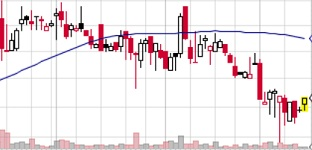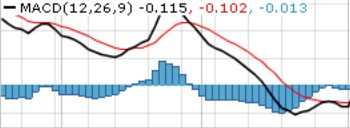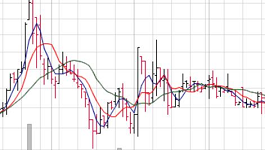# How Does The 100 Day Moving Average Compare To Others?

100-day-moving-average.html

Easy To Understand

The 100 day moving average is a useful tool for any technical investor. However, this is only the starting point. There are many different moving averages.

This is one of the first tools you should learn as well as one of the most common ones. It is simple to understand. That is why investors who are more into fundamental analysis still refer to them every once in a while.

How They Are Calculated?The 100 day moving average takes the closing prices of the day for the last 100 days.

Those numbers are added up and divided by 100. That is done every day. That number gives the price of the moving average for the day. That is how moving averages are created. For different-day moving averages, you just take the average of however many days long the moving average is.

The Different Lengths Of Moving Averages

The longer the days, the slower the moving average will move.

For longer-term trading, two common moving averages that are used in conjunction with one another are 50 and 200. When 50 crosses above 200, it is a buy signal. And vise-versa.

Many short-term traders have found using the 4, 9, and 18 day moving averages together to be quite useful.

The Different Kinds Of Moving Averages

The example I just described is refereed to is known as a simple moving average, or SMA for short.

The four different kinds of moving averages are, linear weighed, exponential, simple, and variable. Out of the four, the simple moving average is the most common. I will go over one more. The second most common one, the…

Exponential Moving Average

The The Exponential Moving Average (EMA) is different from the SMA in a few ways. It is different because the calculation that is used to come up with it is different. So, the circumstances in which you would use it are different as well.

This moving average can be used as a 100 day moving average, as well as any other that you choose.

It was created to get a more fair idea of how a stock is trending. This does not just take the average of the last however many days, like the EMA does.. It goes one step further. It weighs each day differently. The more recent days are weighed more heavily.

Because of this, you will see a quicker reaction when using this moving average than you would with a SMA. This one is not better. It is just different.

Choosing The Appropriate Time For Each Moving Average

There are circumstances when it would be better to use the EMA as opposed to the SMA. and vise-versa.

Just like one type of moving average is not better than the other, one length of one moving average is not better than the length of another.

Every circumstance will be different. You must find out which combination will be the best to use at that time. And, not every stock works well with moving averages. Only the ones that are trending.

Moving Averages Work Best With Trending Stocks

The length of the moving average you decide to use will effect your outcome as far as false indicators.

As I said above, shorter-term moving averages are better for short term trading and longer term moving averages are better for long term trading.

This is because, the shorter you go, the more crossovers you get. The more crossovers you get, the more buy and sell signals you get.

However, even if you are only trading in the short term, its a good idea to have a longer-term moving average open, such as the 100 day moving average. You can look at this to see the general direction that your possible investment is moving.

Use The MACD As A SubstituteIf you do start using more advanced oscillators, you will unintentionally be using moving averages anyways.

One of the most common, and one that I always have open of the various oscillators is the MACD.

This is basically two different moving averages, as well as another moving average showing the difference between the two.

The most common moving averages used with the MACD are the 50 day and 200 day moving averages. However, you can also use the use the 100 day moving average in conjunction with either one of those. But, only two at a time.

With this, you can quickly tell when they cross each other. You may want to use this as a buy or sell signal. I will go over buy and sell signals in just a second.

How Many Moving Averages To Use At One TimeMany people use two moving averages at the same time.

You can use one as well, such as the 100 day moving average. However, with this method, you will get more crossovers because crossovers will take place every time the stock price moves over the moving average.

Using two moving averages is kinda the middle ground. With two, the crossovers are determined when the shorter moving average crosses over the longer one.

With three, you just have more of a warning of a trend reversal. Lets say you use some common numbers: 4, 8, and 18.

When 4 crosses over 8, that is considered an alert. When 8 crosses over 18, that is a signal. This is good if you are trading more based on moving averages. However, myself as well as many other traders use moving averages as just another tool when researching stocks.